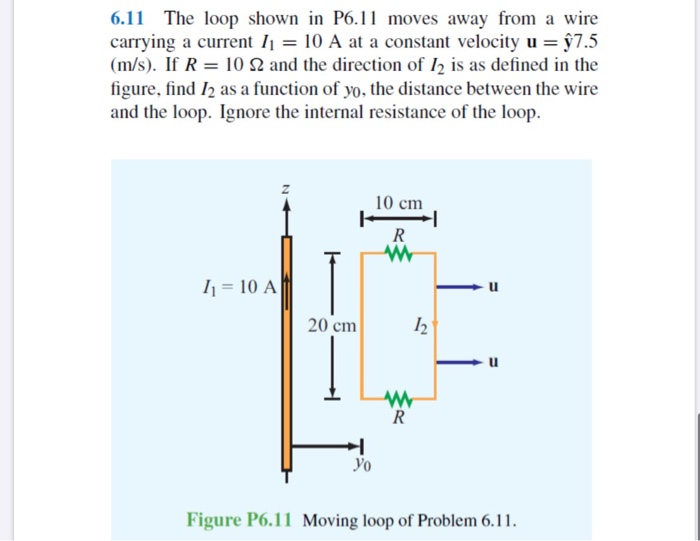# 6.11 The loop shown in P6.11 moves away from a wire carrying a current I1 = 10 A at a constant velocity u = §7.5 (m/s). If R = 10 N and the direction of I2 is as defined in the figure, find I2 as a function of yo, the distance between the wire and the loop. Ignore the internal resistance of the loop. 10 cm I1 = 10 A 20 cm Уo

Questionhelp_outlineImage Transcriptionclose6.11 The loop shown in P6.11 moves away from a wire carrying a current I1 = 10 A at a constant velocity u = §7.5 (m/s). If R = 10 N and the direction of I2 is as defined in the figure, find I2 as a function of yo, the distance between the wire and the loop. Ignore the internal resistance of the loop. 10 cm I1 = 10 A 20 cm Уo fullscreen

### Want to see this answer and more?

Experts are waiting 24/7 to provide step-by-step solutions in as fast as 30 minutes!*

*Response times vary by subject and question complexity. Median response time is 34 minutes and may be longer for new subjects.
Tagged in
SciencePhysics

### Current Electricity